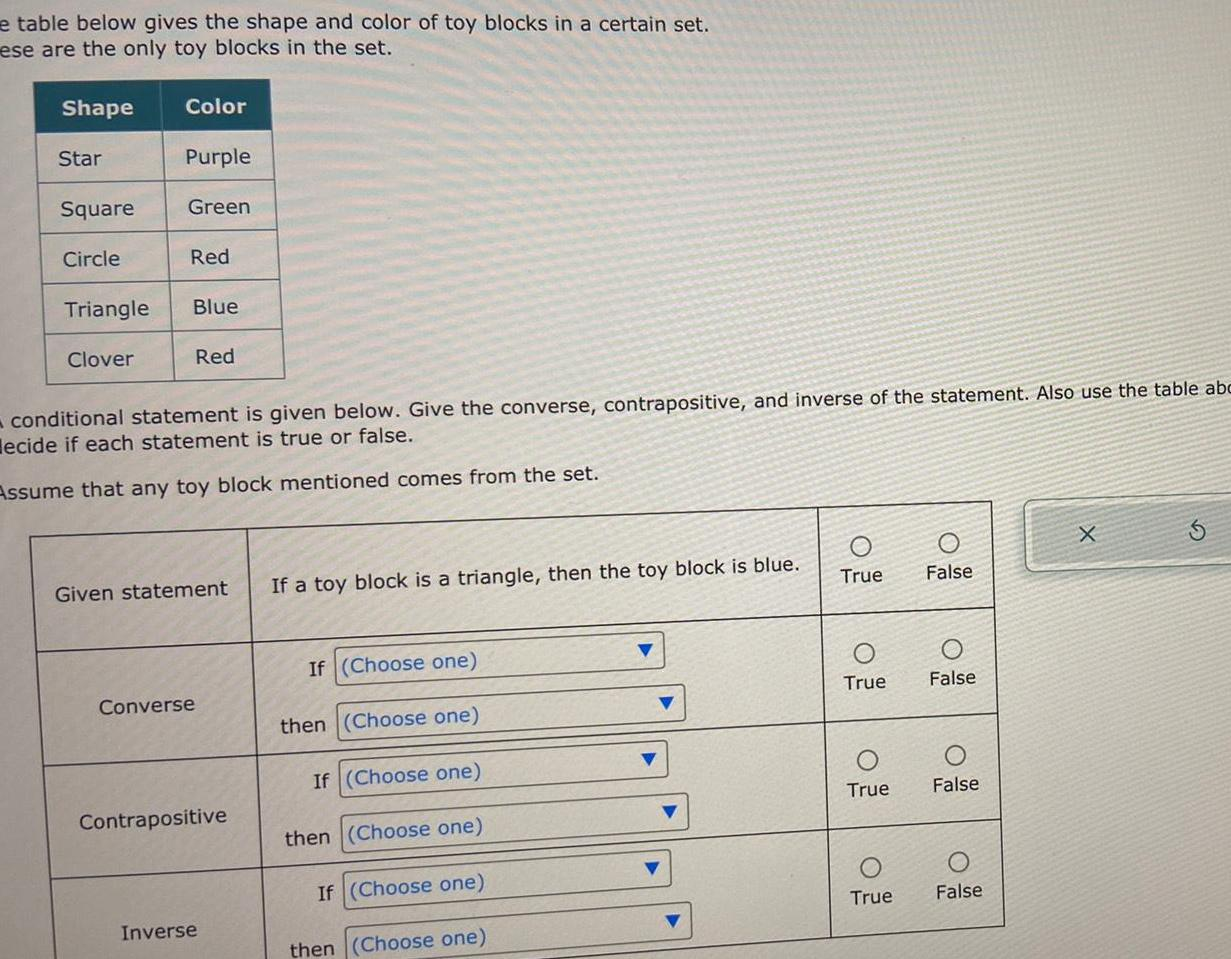Question:

# e table below gives the shape and color of toy blocks in a

Last updated: 9/8/2023e table below gives the shape and color of toy blocks in a certain set ese are the only toy blocks in the set Shape Star Square Circle Triangle Clover Color Purple Green Red Blue Red conditional statement is given below Give the converse contrapositive and inverse of the statement Also use the table abc ecide if each statement is true or false Assume that any toy block mentioned comes from the set Given statement If a toy block is a triangle then the toy block is blue Converse Contrapositive Inverse If Choose one then Choose one If Choose one then Choose one If Choose one then Choose one O True True True True False False False False X